Courses

# Summary - Resultant and Equilibrium of Force System (Non - Coplanar) Mechanical Engineering Notes | EduRev

## Mechanical Engineering : Summary - Resultant and Equilibrium of Force System (Non - Coplanar) Mechanical Engineering Notes | EduRev

The document Summary - Resultant and Equilibrium of Force System (Non - Coplanar) Mechanical Engineering Notes | EduRev is a part of the Mechanical Engineering Course Engineering Mechanics - Notes, Videos, MCQs & PPTs.
All you need of Mechanical Engineering at this link: Mechanical Engineering

Representation of Force

There are two ways of representation of force. The method used depends on the type of problem being solved and the easiest approach to finding a solution.

1. Scalar Notation

2. Vector Notation

Vector Notation Of Forces

1. Two Dimensional Force Systems (Coplanar Forces) It is also possible to represent the x and y components of a force in terms of Cartesian unit vectors i and j.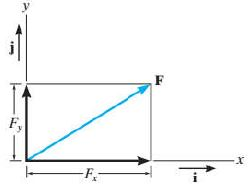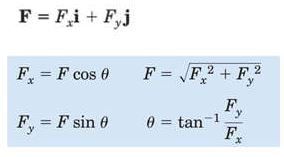where the scalars Fx and Fy are the x and y scalar components of the vector F.

2. Three Dimensional Force Systems(Non-Coplanar Forces)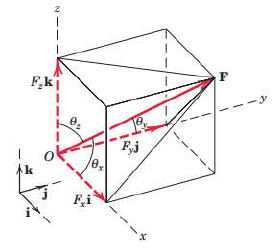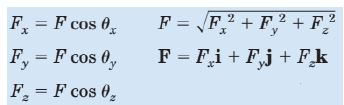Unit Vector Vectors having unit magnitude and represents only the direction of vectors is called a unit vector. It is usually denoted by n.A vector V may be expressed mathematically by multiplying its magnitude V by a vector n whose magnitude is one and whose direction coincides with that of V.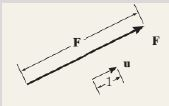The unit vectors along the Rectangular Coordinate axis x, y and z are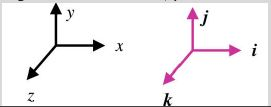PROBLEMS

1. A force vector F = 700i + 1500j is applied to a bolt. Determine the magnitude of force and the angle it forms with the horizontal.

2. A force of 500 N forms angles 600, 450 and 1200 respectively with x, y and z axes. Write the vector form of force.

 Position Vector A position vector r is defined as a fixed vector which locates a point in space relative to another point. a) Position Vector of P relative to origin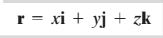b) Position vector of B with respect to A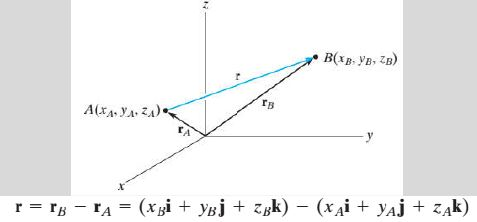A. RESULTANT OF NON COPLANAR FORCES -By Vector Notation

1. Resultant of Non Coplanar Concurrent Forces

In vector notation, the scalar components of the resultant vector can be obtained by adding algebraically the sum of the corresponding scalar components of the force vectors.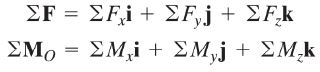where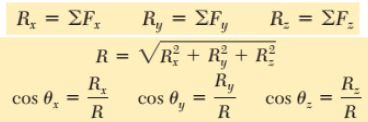B. MOMENT AND COUPLE – In Vector Notation

Moment

Moment is a vector quantity whose direction is perpendicular to the plane of the body. The right-hand rule is used to establish the sense of direction of moment.
Vector Formulation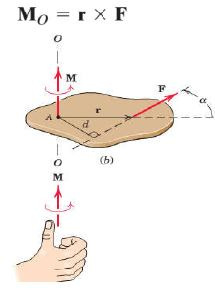Varignon’s Theorem

Vector Formulation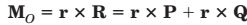Couple

Vector Formulation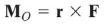Couple vectors are free vectors, i.e., the point of application is not significant.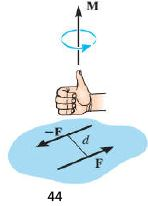Note : Cross Product of vectors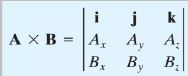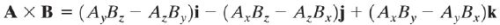2. Resultant of Non Coplanar Non-Concurrent ForcesPROBLEMS1. A table exerts the four forces shown on the floor surface. Reduce the force system to a force– couple system at point O. Determine the resultant of the following force and its location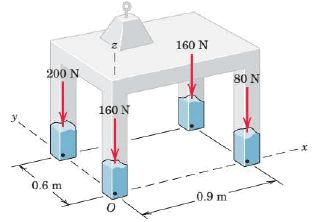2. Replace the two forces acting on the post by a resultant force and couple moment at point O.Express the results in Cartesian vector form.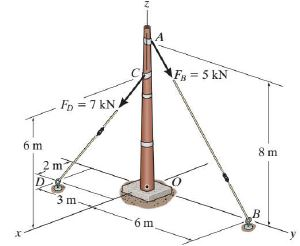C. EQUILIBRIUM OF NON COPLANAR FORCES – By Vector Notation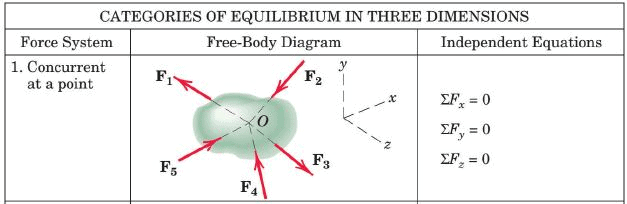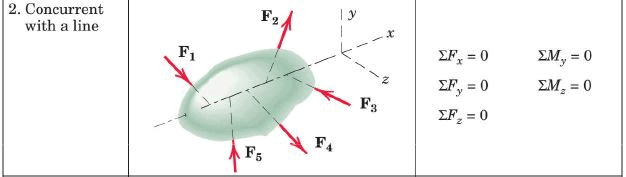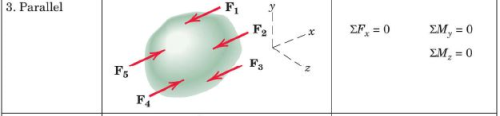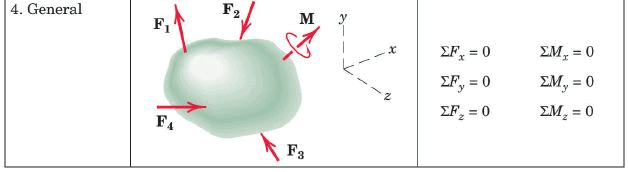1. Equilibrium of Non-Coplanar Concurrent Forces

In vector notation, the equation of equilibrium can be summarized as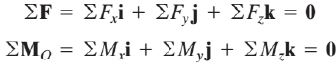Offer running on EduRev: Apply code STAYHOME200 to get INR 200 off on our premium plan EduRev Infinity!

,

,

,

,

,

,

,

,

,

,

,

,

,

,

,

,

,

,

,

,

,

;